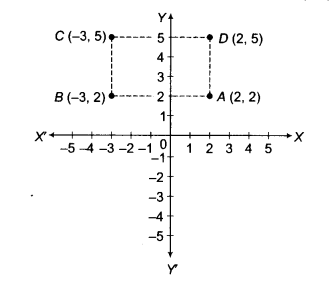# The three vertices of a rectangle ABCD are A(2, 2), B{-3,2) and C(-3,5)

The three vertices of a rectangle ABCD are A(2, 2), B{-3,2) and C(-3,5). Plot these points on a graph paper and find the area of rectangle ABCD.

Given, three vertices of rectangle ABCD are A{2,2), B(-3,2) and C(-3, 5).
Now, plotting the points on the graph paper, as shown below, we find that the coordinates of D are (2, 5).Then, AB = 5 units and BC = 3 units
∴ Area of a rectangle = Length x Breadth
= 3x5 = 15sq units